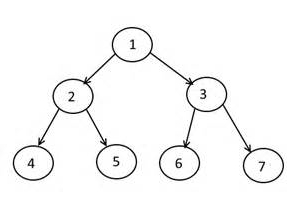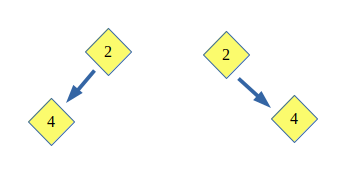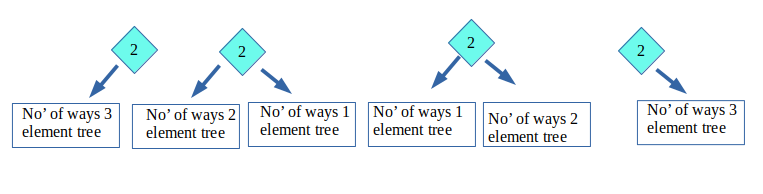# Number of Binary Trees for given Preorder Sequence length

Count the number of Binary Tree possible for a given Preorder Sequence length n.

Examples:

```Input : n = 1
Output : 1

Input : n = 2
Output : 2

Input : n = 3
Output : 5
```

## Recommended: Please try your approach on {IDE} first, before moving on to the solution.

Background :

In Preorder traversal, we process the root node first, then traverse the left child node and then right child node.

For example preorder traversal of below tree is 1 2 4 5 3 6 7Finding number of trees with given Preorder:

Number of Binary Tree possible if such a traversal length (let’s say n) is given.

Let’s take an Example : Given Preorder Sequence –> 2 4 6 8 10 (length 5).

• Assume there is only 1 node (that is 2 in this case), So only 1 Binary tree is Possible
• Now, assume there are 2 nodes (namely 2 and 4), So only 2 Binary Tree are Possible:
•• Now, when there are 3 nodes (namely 2, 4 and 6), So Possible Binary tree are 5
•NOTE* Since we have already calculated for 1, 2 and 3 nodes. We don’t need to evaluate them again for successive nodes.

• Consider 4 nodes (that are 2, 4, 6 and 8), So Possible Binary Tree are 14.
Let’s say BT(1) denotes number of Binary tree for 1 node. (We assume BT(0)=1)
BT(4) = BT(0) * BT(3) + BT(1) * BT(2) + BT(2) * BT(1) + BT(3) * BT(0)
BT(4) = 1 * 5 + 1 * 2 + 2 * 1 + 5 * 1 = 14
•• Similarly, considering all the 5 nodes (2, 4, 6, 8 and 10). Possible number of Binary Tree are:
BT(5) = BT(0) * BT(4) + BT(1) * BT(3) + BT(2) * BT(2) + BT(3) * BT(1) + BT(4) * BT(0)
BT(5) = 1 * 14 + 1 * 5 + 2 * 2 + 5 * 1 + 14 * 1 = 42Hence, Total binary Tree for Pre-order sequence of length 5 is 42.

We use Dynamic programming to calculate the possible number of Binary Tree. We take one node at a time and calculate the possible Trees using previously calculated Trees.

## C++

 `// C++ Program to count possible binary trees ` `// using dynamic programming ` `#include ` `using` `namespace` `std; ` ` `  `int` `countTrees(``int` `n) ` `{ ` `    ``// Array to store number of Binary tree ` `    ``// for every count of nodes ` `    ``int` `BT[n + 1]; ` `    ``memset``(BT, 0, ``sizeof``(BT)); ` ` `  `    ``BT = BT = 1; ` ` `  `    ``// Start finding from 2 nodes, since ` `    ``// already know for 1 node. ` `    ``for` `(``int` `i = 2; i <= n; ++i)  ` `        ``for` `(``int` `j = 0; j < i; j++) ` `            ``BT[i] += BT[j] * BT[i - j - 1]; ` ` `  `    ``return` `BT[n]; ` `} ` ` `  `// Driver code ` `int` `main() ` `{ ` `    ``int` `n = 5; ` `    ``cout << ``"Total Possible Binary Trees are : "` `        ``<< countTrees(n) << endl; ` `    ``return` `0; ` `} `

## Java

 `// Java Program to count  ` `// possible binary trees ` `// using dynamic programming ` `import` `java.io.*; ` ` `  `class` `GFG ` `{ ` `static` `int` `countTrees(``int` `n) ` `{ ` `    ``// Array to store number ` `    ``// of Binary tree for  ` `    ``// every count of nodes ` `    ``int` `BT[] = ``new` `int``[n + ``1``]; ` `    ``for``(``int` `i = ``0``; i <= n; i++) ` `    ``BT[i] = ``0``; ` `    ``BT[``0``] = BT[``1``] = ``1``; ` ` `  `    ``// Start finding from 2 ` `    ``// nodes, since already  ` `    ``// know for 1 node. ` `    ``for` `(``int` `i = ``2``; i <= n; ++i)  ` `        ``for` `(``int` `j = ``0``; j < i; j++) ` `            ``BT[i] += BT[j] * ` `                     ``BT[i - j - ``1``]; ` ` `  `    ``return` `BT[n]; ` `} ` ` `  `// Driver code ` `public` `static` `void` `main (String[] args)  ` `{ ` `int` `n = ``5``; ` `System.out.println(``"Total Possible "` `+  ` `                ``"Binary Trees are : "` `+  ` `                       ``countTrees(n)); ` `} ` `} ` ` `  `// This code is contributed by anuj_67. `

## Python3

 `# Python3 Program to count possible binary  ` `# trees using dynamic programming  ` ` `  `def` `countTrees(n) : ` ` `  `    ``# Array to store number of Binary  ` `    ``# tree for every count of nodes  ` `    ``BT ``=` `[``0``] ``*` `(n ``+` `1``)  ` ` `  `    ``BT[``0``] ``=` `BT[``1``] ``=` `1` ` `  `    ``# Start finding from 2 nodes, since  ` `    ``# already know for 1 node.  ` `    ``for` `i ``in` `range``(``2``, n ``+` `1``):  ` `        ``for` `j ``in` `range``(i): ` `            ``BT[i] ``+``=` `BT[j] ``*` `BT[i ``-` `j ``-` `1``]  ` ` `  `    ``return` `BT[n]  ` ` `  `# Driver Code  ` `if` `__name__ ``=``=` `'__main__'``: ` ` `  `    ``n ``=` `5` `    ``print``(``"Total Possible Binary Trees are : "``,  ` `                                 ``countTrees(n)) ` `                                  `  `# This code is contributed by ` `# Shubham Singh(SHUBHAMSINGH10) `

## C#

 `// C# Program to count  ` `// possible binary trees ` `// using dynamic programming ` `using` `System; ` ` `  `class` `GFG ` `{ ` `static` `int` `countTrees(``int` `n) ` `{ ` `    ``// Array to store number ` `    ``// of Binary tree for  ` `    ``// every count of nodes ` `    ``int` `[]BT = ``new` `int``[n + 1]; ` `    ``for``(``int` `i = 0; i <= n; i++) ` `        ``BT[i] = 0; ` `        ``BT = BT = 1; ` ` `  `    ``// Start finding from 2 ` `    ``// nodes, since already  ` `    ``// know for 1 node. ` `    ``for` `(``int` `i = 2; i <= n; ++i)  ` `        ``for` `(``int` `j = 0; j < i; j++) ` `            ``BT[i] += BT[j] * ` `                     ``BT[i - j - 1]; ` ` `  `    ``return` `BT[n]; ` `} ` ` `  `// Driver code ` `static` `public` `void` `Main (String []args)  ` `{ ` `    ``int` `n = 5; ` `    ``Console.WriteLine(``"Total Possible "` `+  ` `                      ``"Binary Trees are : "` `+  ` `                             ``countTrees(n)); ` `} ` `} ` ` `  `// This code is contributed  ` `// by Arnab Kundu `

## PHP

 ` `

Output:

```Total Possible Binary Trees are : 42
```

Alternative :
This can also be done using Catalan number Cn = (2n)!/(n+1)!*n!

For n = 0, 1, 2, 3, … values of Catalan numbers are 1, 1, 2, 5, 14, 42, 132, 429, 1430, 4862, …. So are numbers of Binary Search Trees.

This article is contributed by Shubham Rana. If you like GeeksforGeeks and would like to contribute, you can also write an article using contribute.geeksforgeeks.org or mail your article to contribute@geeksforgeeks.org. See your article appearing on the GeeksforGeeks main page and help other Geeks.

GeeksforGeeks has prepared a complete interview preparation course with premium videos, theory, practice problems, TA support and many more features. Please refer Placement 100 for details

My Personal Notes arrow_drop_up

Article Tags :
Practice Tags :

8

Please write to us at contribute@geeksforgeeks.org to report any issue with the above content.